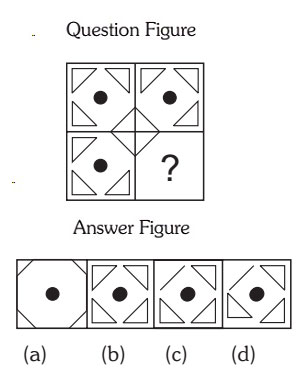## Completion Of Incomplete Pattern

#### Completion Of Incomplete Pattern

Direction: In each of the following questions, find out which of the answer figures (a), (b), (c) and (d) completes the figure?

1. Following Question has four alternatives, among which one completes the figures.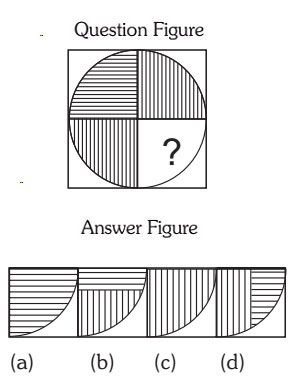1. Figures are moving 90 degree in clockwise direction.

##### Correct Option: A

Figures are moving 90 degree in clockwise direction. 1st figure moves 90 degree in clockwise direction then 2nd figure also moves 90 degree in clockwise direction. similarly 3rd figure will also move 90 degree in clockwise direction.

1. Select the correct figure from the given Answer Figure that would complete the Question figure.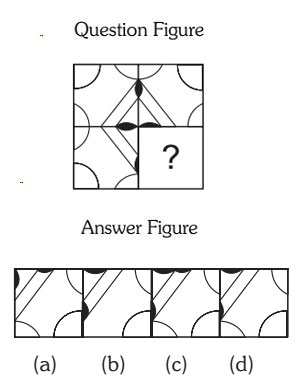##### Correct Option: D

1. Following Question has four alternatives, among which one completes the figures.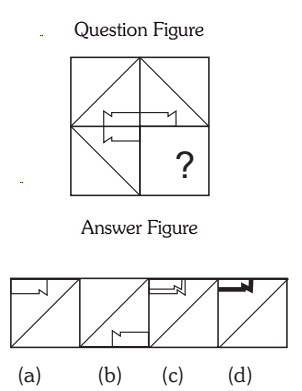##### Correct Option: A

1. Select a suitable figure from the four alternatives that would complete the figure.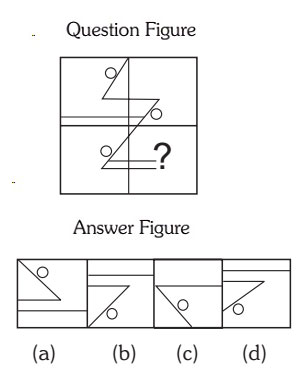##### Correct Option: B

1. Select a suitable figure from the four alternatives that would complete the figure.